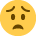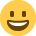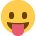Young Carers of The Mix, we need you! Tell us about your experiences of our services and give yourself a chance to win £50 of Amazon vouchers.
Want to tell us about your experiences using The Mix and be in with a chance of winning a £200 Amazon Voucher? Click here to go to our survey!

# Homework help... Again

Posts: 1,876,328 The Mix Honorary Guru
Have a test tomorrow and going through worksheets which hold the same kind of questions as my test.

Have forgotten so much of the stuff from last year and I am seriously screwed with this.
Help to solve these questions would be dearly appericiated, you have no clue.

1.
If I get told to solve the equation: log(x+1) + log(x-2) = 1, then how the hell do I even start?

2.
How do I solve the equation: 3000=167952,882*x^-2,017?

3.
How do I solve the equation: 90=1117,74*x^-0,805?

So basically I am mostly screwed with log and polynomials/power (that's what the dictionary says it's called in English).

Please somebody help·

• Posts: 1,876,328 The Mix Honorary Guru
Re: Homework help... Again
Originally posted by Jacqueline the Ripper

1.
If I get told to solve the equation: log(x+1) + log(x-2) = 1, then how the hell do I even start?

2.
How do I solve the equation: 3000=167952,882*x^-2,017?

3.
How do I solve the equation: 90=1117,74*x^-0,805?

So basically I am mostly screwed with log and polynomials/power (that's what the dictionary says it's called in English).

Please somebody helpJaqu shall I entertain you till a genius arrives cos I got lost soon as you mentioned test·
• Posts: 1,876,328 The Mix Honorary Guru
1. Take the exponential of both sides (I'm assuming these are natural logarithms, if they are not raise them to the power of the log eg 10^{...} for a base 10 logarithm):

exp{log(x+1) + log(x+2)} = exp {1}

Then use the laws of powers (exp{a+b} = exp{a}*exp{b})to separate out the left hand side:

exp{log(x+1)}*exp{log(x+2)} = exp {1}

Generally exp{log(y)} = y if y>0, so applying this rule:

(x+1)*(x+2) = exp {1} [note condition that x>2]

Then expand out the factors and solve the quadratic for x!

At least I think that lot is right2 and 3. Remember this simple rule "whatever you do to one side of the equation, you must also do to the other. For 2:

3000=167952,882*x^-2,017

Add 2,017 to both sides:

3000 + 2,017 = 167952,882*x

Then divide both sides by 167952,882:

3002,017 / 167952,882 = x

And do a similar thing for 3.

Hope that helps·
• Posts: 1,876,328 The Mix Honorary Guru
Bristolmike can you help me do my stats for my masters then!! hehe!

I failed the assignment but no surprsies as I have no idea what I'm doing! Think I will need to google to find help (not cheat I mean helpful explanations as the uni text books are pants!)
·
• Posts: 1,876,328 The Mix Honorary Guru
Originally posted by Bristolmike
1. Take the exponential of both sides (I'm assuming these are natural logarithms, if they are not raise them to the power of the log eg 10^{...} for a base 10 logarithm):

exp{log(x+1) + log(x+2)} = exp {1}

Then use the laws of powers (exp{a+b} = exp{a}*exp{b})to separate out the left hand side:

exp{log(x+1)}*exp{log(x+2)} = exp {1}

Generally exp{log(y)} = y if y>0, so applying this rule:

(x+1)*(x+2) = exp {1} [note condition that x>2]

Then expand out the factors and solve the quadratic for x!

At least I think that lot is right2 and 3. Remember this simple rule "whatever you do to one side of the equation, you must also do to the other. For 2:

3000=167952,882*x^-2,017

Add 2,017 to both sides:

3000 + 2,017 = 167952,882*x

Then divide both sides by 167952,882:

3002,017 / 167952,882 = x

And do a similar thing for 3.

Hope that helps:eek2:
·
• Posts: 1,876,328 The Mix Honorary Guru
Originally posted by lucifer devil
:eek2:
Thats what I was going to say/do
So I'll use this one instead:crazyeyes
·
• Posts: 1,876,328 The Mix Honorary Guru
no more maths, keep it away, bad evil maths ARRRRRRRRRRRRRRRRRRRRRRRRRRRRRRRRRRRRRR

Bopz :crazyeyes
·
• Posts: 1,876,328 The Mix Honorary Guru
Thanks bristolmikeThough I don't think that the last calculation is correct. Don't have the strength to point it out now. Still have a lot of math to go through before tomorrow :crazyeyes
·
• Posts: 1,876,328 The Mix Honorary Guru
Originally posted by Jowolvo
Bristolmike can you help me do my stats for my masters then!! hehe!

I failed the assignment but no surprsies as I have no idea what I'm doing! Think I will need to google to find help (not cheat I mean helpful explanations as the uni text books are pants!)

Depends, I aint that hot on stats!
·
• Posts: 1,876,328 The Mix Honorary Guru
Originally posted by Jacqueline the Ripper
Thanks bristolmikeThough I don't think that the last calculation is correct. Don't have the strength to point it out now. Still have a lot of math to go through before tomorrow :crazyeyes

Nuts, you're quite right, I missed the fact that they are raised to a power!

Just ignore the addition bit....divide both sides by the 167952,882 and then take the -2,017th root of that (or raise both sides to the power 1/2,017 and invert the lot)...thus:

3000 / 167952,882 = x^-2,017

then

(3000 / 167952,882)^(1 / 2,017) = 1 / x = x^-1

Then just take 1/ answer to get x (I get x = 7.356 to 3 decimal places).

Similarly for 3.
·
• Posts: 1,876,328 The Mix Honorary Guru
Exactly. Just to laxy to write it all myselfOff to shower, remembering forms, and bed.

People wish me luck. I need it. Seriously.
·
• Posts: 1,876,328 The Mix Honorary Guru
Originally posted by Bristolmike
Nuts, you're quite right, I missed the fact that they are raised to a power!
Ah, of course! Now it makes much more sense!:yeees:
·
• Posts: 1,876,328 The Mix Honorary Guru
Did you hear that noise? That "Whooosh!" sound? The sound of all of that going right over my head.I just don't get maths. It's like some bizarre foreign language to me. Every time I even think I get close to understanding it, it suddenly makes absolutely no sense.·
• Posts: 1,876,328 The Mix Honorary Guru
It's wierd, I've always been better at numbers than I have bin at lanwage (deliberate and obvious I know, but funny none the less!). In my experience people generally fall into two types: those good at science and numbers and those good at the arts. I would be the former. I guess I don't see number like a poet sees words, it's just that I've been dealing with them so long and to such a relatively advanced level compared to the stuff in this thread that the rules governing numbers have become second nature to me.

That's all it really is after all: a set of rules and methods you have to learn. Once you learn all the rules and practice the methods for solving problems, you can approach any mathematical problem with the confidence to solve it. Like learning a languge; at first you learn some basic words and phrases to get by and eventually you get to a stage where you correct for things like correct tense and voice. It's similar in math and science. At first you learn over simplified truths but eventually you deal in precision and can handle completely alien problems. That's what I think anyway J and Mr_Wobble.
·
• Posts: 1,876,328 The Mix Honorary Guru
I know that's how it's supposed to work Bristolmike, but it just gets to a point where I get lost, and don't understand anymore. I think it's a case of trying to learn too much too quickly, and not getting the hang of some of the basics before moving on. When I went to Uni I could hardly understand anything the Maths tutor was saying, it was all gobbledygook to me.·
• Posts: 1,876,328 The Mix Honorary Guru
It's not an either or regarding sciences and art/humanistics.

I think to be honest that it's about interest. At least that's how it i with me. When i can apply science to something useful I don't have problems. Otherwise it plain bores me and I really can't bother.
When looking at my grades in school it's very noticable what has my interest and what hasn't.
·
• Posts: 1,876,328 The Mix Honorary Guru
Originally posted by Bristolmike
2 and 3. Remember this simple rule "whatever you do to one side of the equation, you must also do to the other. For 2:

3000=167952,882*x^-2,017

Add 2,017 to both sides:

3000 + 2,017 = 167952,882*x

Then divide both sides by 167952,882:

3002,017 / 167952,882 = x

And do a similar thing for 3.

Hope that helpsnah man your a bit wrong it isnt x-2017 but x^-2017 so you need to do logs of both sides (if u need help with log laws, ask!)
log3000=log(167952,882*x^-2,017)
log3000=log167952,882 + log(x^-2,017)
log3000 - log167952,882 = -2017*log(x)
[ log (3000/167952,882) ] / -2017 = log x
then whatever you get for the left hand side of that, do inverse log, and you have x
and you can use either the natural log (ln) or log(base 10), just use it throughout, and use the same inverse log function!

<doing a stupidly hard maths course for 1st yr uni chemistry with maths, so this sint too much of a challenge, just dont ask me matrices questions!!!!>
·
• Posts: 1,876,328 The Mix Honorary Guru
Logs was just one thing I did not get at all at higher... What the hell are they anyway?! And yeah, there does seem to be two camps of people. Those good at Maths and those good at English. I'm the latter one (well, occasionally anyway...)
·